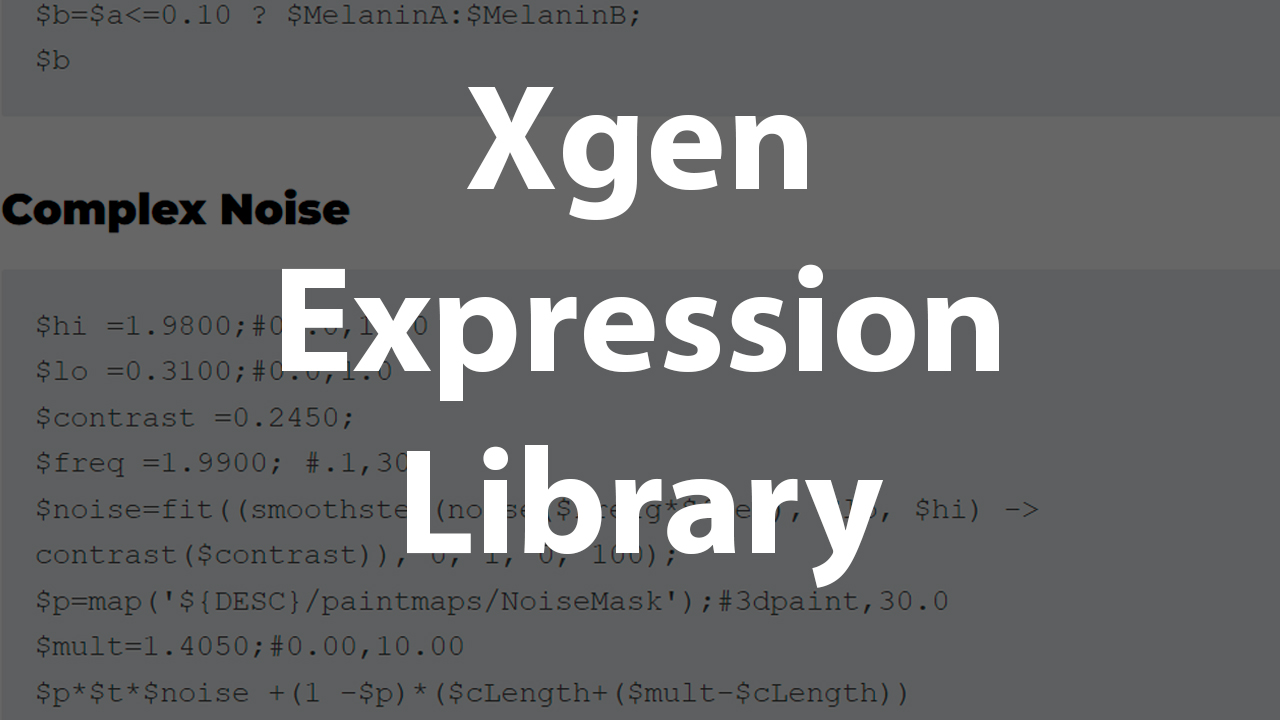January 31, 2023

# Xgen Expression Library#### Randon Value

\$randmin = 0.001; #0.000,0.050 \$randMax = 0.003; #0.000,0.050 \$seed = 154.0000; \$randValue = rand(\$randmin,\$randMax,\$seed); \$randValue

#### Randon by absolute Length ( a must for the cut modifier)

\$randmin = 0.001; #0.000,0.050 \$randMax = 0.003; #0.000,0.050 \$seed = 154.0000; \$randValue = rand(\$randmin,\$randMax,\$seed); \$randValue*@cLength

#### Percentage

\$percentage = 0.1500; #0.000,1.000 \$randValue = rand(0,1)<\$percentage; \$randValue

#### Melanin Color

\$percentStray=51;#0,100 \$a=rand() < \$percentStray/100.0 ? 1 : 0; \$MelaninA=0.15; \$MelaninB=0.65; \$b=\$a<=0.10 ? \$MelaninA:\$MelaninB; \$b

#### Complex Noise

\$hi =1.9800;#01.0,10.0 \$lo =0.3100;#0.0,1.0 \$contrast =0.2450; \$freq =1.9900; #.1,30 \$noise=fit((smoothstep(noise(\$Prefg*\$freq), \$lo, \$hi) -> contrast(\$contrast)), 0, 1, 0, 100); \$p=map('\${DESC}/paintmaps/NoiseMask');#3dpaint,30.0 \$mult=1.4050;#0.00,10.00 \$p*\$t*\$noise +(1 -\$p)*(\$cLength+(\$mult-\$cLength))

#### Ramp per length ( \$t not absolute length)

\$ramp=linearstep(\$t,0.0,1); \$ramp

#### Random per length ( \$t )

\$randmin = 0.7000; #0.000,0.050 \$randMax = 1.0000; #0.000,0.050 \$seed = 154.0000; \$randValue = rand(\$randmin,\$randMax,\$seed); \$value = linearstep(\$randValue,0,1); \$value

#### Displacement Re Fit

\$a=disp(); \$min=0.1700; \$max=1.0000;#0.0,5.0 fit(\$a, 0,1, \$min, \$max*2)

#### Flyaway percentage

By Mustafa Mohsen

\$FlyawayPercentage =0;#0,100 \$FlyawayAmount=0.0000;#0.00,1.00 \$FlyawayAmountMap=map('\${DESC}/paintmaps/FlyawayAmount_map');#3dpaint,10.0 (rand() > \$FlyawayPercentage/100.0 ? 1 : 0)+\$FlyawayAmount+\$FlyawayAmountMap

#### Operation by length ( Change * for any of + - / when needed)

\$mult = 9.40141;#0.00,10.00 \$mult*\$cLength